##### Calculus Workbook For Dummies with Online PracticeA limit problem asks you to determine what the y value of a function is zeroing in on as the x value approaches a particular number. With your calculator, you can solve a limit problem using graphing mode.

To begin, enter the limit expression in graphing or “y =” mode, go to Table Setup, set Tblstart to the arrow-number, and set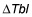to something small like 0.01 or 0.001. When you look at the table, you’ll often see the y values getting closer and closer to the limit answer as x homes in on the arrow-number. If it’s not clear what the y values are approaching, try a smaller increment for thenumber. This method often gives you a good feel for what’s happening in a limit problem.

## Practice questions

1. Evaluate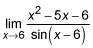2. Use your calculator to determine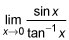1. The answer is 7. Step 1. Enter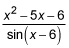in graphing or “y =” mode. Step 2. Go to Table Setup and set tblStart to the arrow-number, 6, andto 0.01. Step 3. Go to the Table and you’ll see the y values getting closer and closer to 7 as you scroll toward x = 6 from above and below 6. So, 7 is your answer.
2. The answer is 1. Enter the function in graphing mode like this: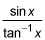Then go to table setup and enter a small increment into(try 0.01 for this problem), and enter the arrow-number, 0, into tblStart. When you scroll through the table near x = 0, you’ll see the y values getting closer and closer to the round number 1. That’s your answer. This problem is not easy to do with algebra.